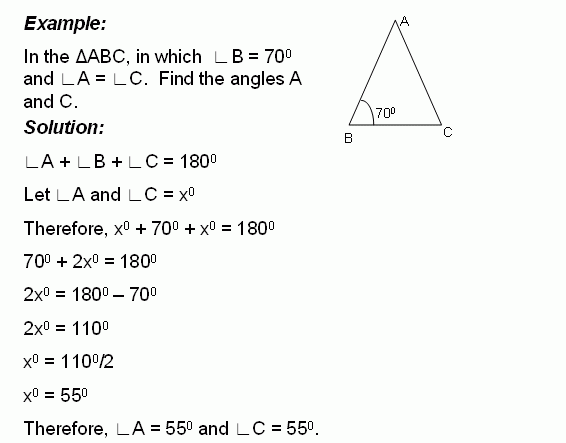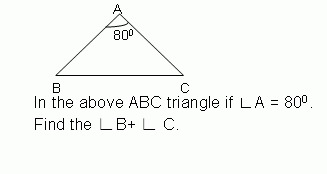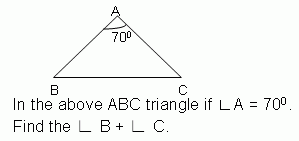Email us to get an instant 20% discount on highly effective K-12 Math & English kwizNET Programs!

#### Online Quiz (WorksheetABCD)

Questions Per Quiz = 2 4 6 8 10

### Middle/High School Algebra, Geometry, and Statistics (AGS)6.14 Find the Angles of TriangleDirections: Solve the following problems. Also write at least 10 examples of your own.
 Q 1:100 degrees75 degrees160 degrees Q 2: Find the angles of the triangle and classify based on the angles.45, 45, 9020, 60, 10030, 60, 90 Q 3:110 degrees100 degrees105 degrees Q 4:60 degrees65 degrees70 degrees Q 5: The measure of angle x is _____ degrees.Answer: Q 6:75 degrees60 degrees65 degrees Q 7:100 degrees90 degrees95 degrees Q 8: Find the angles of the triangle and classify based on the angles.32, 96, right angle triangle26, 64, right angle triangle30, 60, right angle triangle Question 9: This question is available to subscribers only! Question 10: This question is available to subscribers only!